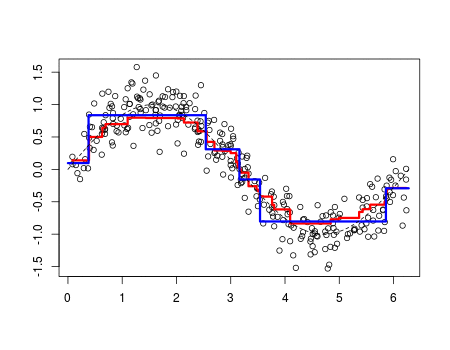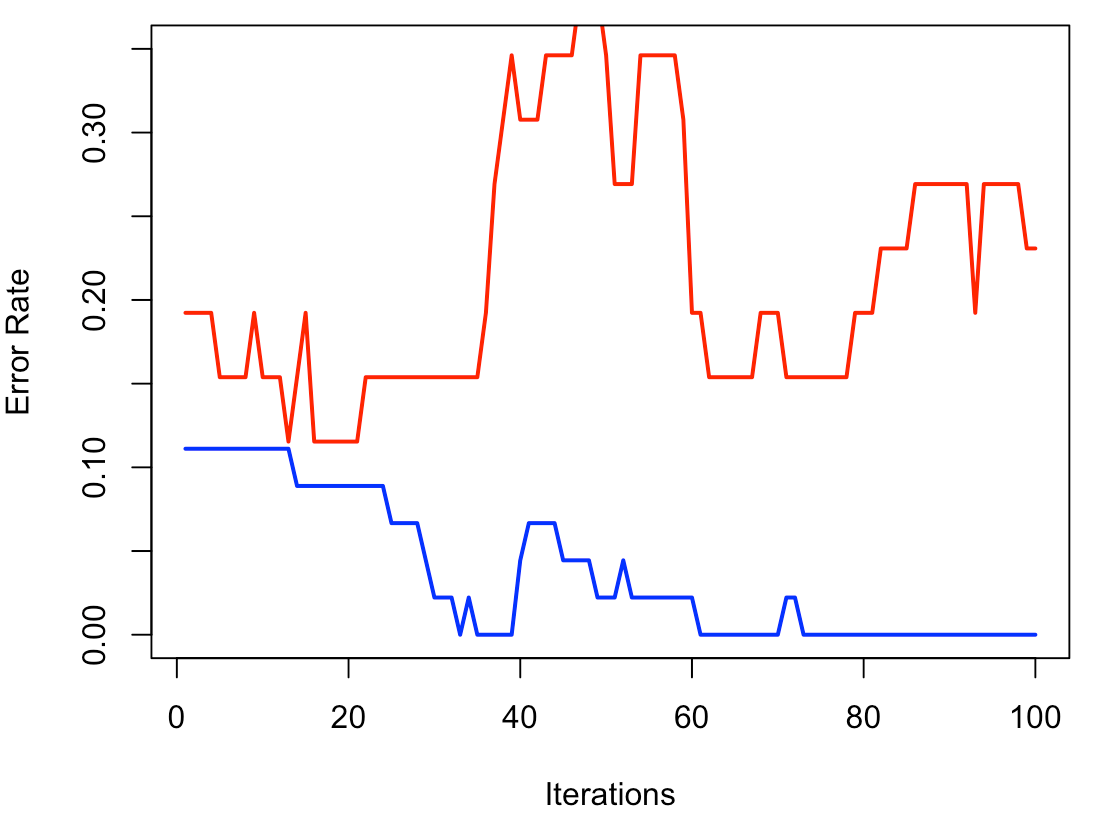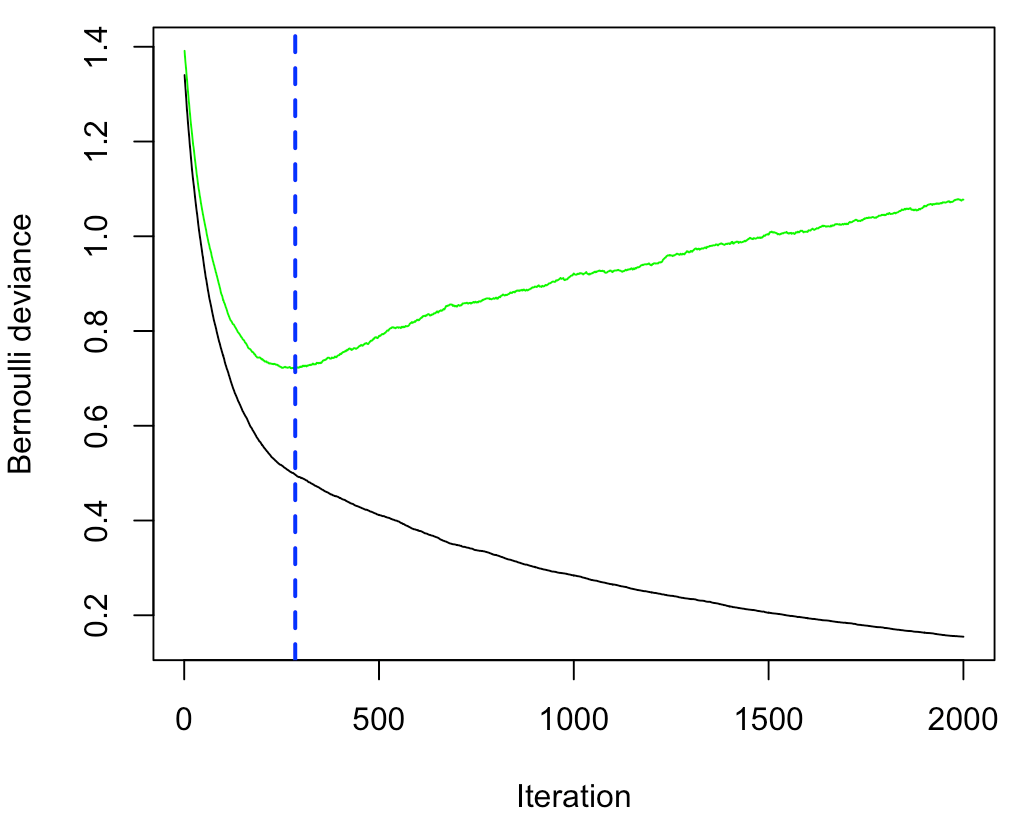# Classification from scratch, boosting 11/8

June 8, 2018
By

Want to share your content on R-bloggers? click here if you have a blog, or here if you don't.

Eleventh post of our series on classification from scratch. Today, that should be the last one… unless I forgot something important. So today, we discuss boosting.

## An econometrician perspective

I might start with a non-conventional introduction. But that’s actually how I understood what boosting was about. And I am quite sure it has to do with my background in econometrics.

The goal here is to solve something which looks like$$m^\star=\underset{m\in\mathcal{M}}{\text{argmin}}\left\lbrace\sum_{i=1}^n \ell(y_i,m(\mathbf{x}_i))\right\rbrace$$for some loss function $\ell$, and for some set of predictors $\mathcal{M}$. This is an optimization problem. Well, optimization is here in a function space, but still, that’s simply an optimization problem. And from a numerical perspective, optimization is solve using gradient descent (this is why this technique is also called gradient boosting). And the gradient descent can be visualized like belowAgain, the optimum is not some some real value $x^\star$, but some function $m^\star$. Thus, here we will have something like$$m^{(k)}=m^{(k-1)}+\underset{h\in\mathcal{H}}{\text{argmin}}\left\lbrace \sum_{i=1}^n \ell(y_i,m^{(k-1)}(\mathbf{x}_i)+h(\mathbf{x}_i))\right\rbrace$$(as they write it is serious articles) where the term on the right can also be written$$m^{(k)}=m^{(k-1)}+\underset{h\in\mathcal{H}}{\text{argmin}}\left\lbrace \sum_{i=1}^n \ell(\underbrace{y_i-m^{(k-1)}(\mathbf{x}_i)}_{\varepsilon_{k,i}},h(\mathbf{x}_i))\right\rbrace$$I prefer the later, because we see clearly that $f$ is some model we fit on the remaining residuals.

We can rewrite it like that: define$$r_{i,k}=-\left.\frac{\partial \ell(y_i,m(\mathbf{x}_i))}{\partial m(\mathbf{x}_i)}\right\vert_{m(\mathbf{x}_i)=m^{(k-1)}(\mathbf{x}_i)}$$for all $i=1,\cdots,n$. The goal is to fit a model so that $r_{i,k}=h^\star(\mathbf{x}_i)$, and when we have that optimal function, set $m_k(\mathbf{x})=m_{k-1}(\mathbf{x})+\gamma_k h^\star(\mathbf{x})$ (yes, we can include some shrinkage here).

Two important comments here. First of all, the idea should be weird to any econometrician. First, we fit a model to explain $y$ by some covariates $\mathbf{x}$. Then consider the residuals $\widehat{\varepsilon}$, and to explain them with the same covariate $\mathbf{x}$. If you try that with a linear regression, you’d done at the end of step 1, since residuals $\widehat{\varepsilon}$ are orthogonal to covariates $\mathbf{x}$: no way that we can learn from them. Here it works because we consider simple non linear model. And actually, something that can be used is to add a shrinkage parameter. Do not consider $\widehat{\varepsilon}=y-\widehat{m}(\mathbf{x})$ but $\widehat{\varepsilon}=y-\gamma\widehat{m}(\mathbf{x})$. The idea of weak learners is extremely important here. The more we shrink, the longer it will take, but that’s not (too) important.

I should also mention that it’s nice to keep learning from our mistakes. But somehow, we should stop, someday. I said that I will not mention this part in this series of posts, maybe later on. But heuristically, we should stop when we start to overfit. And this can be observed either using a split training/validation of the initial dataset or to use cross validation. I will get back on that issue later one in this post, but again, those ideas should probably be dedicated to another series of posts.

## Learning with splines

Just to make sure we get it, let’s try to learn with splines. Because standard splines have fixed knots, actually, we do not really “learn” here (and after a few iterations we get to what we would have with a standard spline regression). So here, we will (somehow) optimize knots locations. There is a package to do so. And just to illustrate, use a Gaussian regression here, not a classification (we will do that later on). Consider the following dataset (with only one covariate)

 1 2 3 4 5  n=300 set.seed(1) u=sort(runif(n)*2*pi) y=sin(u)+rnorm(n)/4 df=data.frame(x=u,y=y)

For an optimal choice of knot locations, we can use

 1 2  library(freeknotsplines) xy.freekt=freelsgen(df$x, df$y, degree = 1, numknot = 2, 555)

With 5% shrinkage, the code it simply the following

 1 2 3 4 5 6 7 8 9 10 11 12 13 14 15 16 17 18 19 20 21 22 23  v=.05 library(splines) xy.freekt=freelsgen(df$x, df$y, degree = 1, numknot = 2, 555) fit=lm(y~bs(x,degree=1,knots=xy.freekt@optknot),data=df) yp=predict(fit,newdata=df) df$yr=df$y - v*yp YP=v*yp for(t in 1:200){ xy.freekt=freelsgen(df$x, df$yr, degree = 1, numknot = 2, 555) fit=lm(yr~bs(x,degree=1,knots=xy.freekt@optknot),data=df) yp=predict(fit,newdata=df) df$yr=df$yr - v*yp YP=cbind(YP,v*yp)} nd=data.frame(x=seq(0,2*pi,by=.01)) viz=function(M){ if(M==1) y=YP[,1] if(M>1) y=apply(YP[,1:M],1,sum) plot(df$x,df$y,ylab="",xlab="") lines(df$x,y,type="l",col="red",lwd=3) fit=lm(y~bs(x,degree=1,df=3),data=df) yp=predict(fit,newdata=nd) lines(nd$x,yp,type="l",col="blue",lwd=3) lines(nd$x,sin(nd$x),lty=2)}

To visualize the ouput after 100 iterations, use

 1  viz(100)Clearly, we see that we learn from the data here… Cool, isn’t it?

## Learning with stumps (and trees)

Let us try something else. What if we consider at each step a regression tree, instead of a linear-by-parts regression (that was considered with linear splines).

 1 2 3 4 5 6 7 8 9 10 11  library(rpart) v=.1 fit=rpart(y~x,data=df) yp=predict(fit) df$yr=df$y - v*yp YP=v*yp for(t in 1:100){ fit=rpart(yr~x,data=df) yp=predict(fit,newdata=df) df$yr=df$yr - v*yp YP=cbind(YP,v*yp)}

Again, to visualise the learning process, use

 1 2 3 4 5 6 7 8  viz=function(M){ y=apply(YP[,1:M],1,sum) plot(df$x,df$y,ylab="",xlab="") lines(df$x,y,type="s",col="red",lwd=3) fit=rpart(y~x,data=df) yp=predict(fit,newdata=nd) lines(nd$x,yp,type="s",col="blue",lwd=3) lines(nd$x,sin(nd$x),lty=2)}This time, with those trees, it looks like not only we have a good model, but also a different model from the one we can get using a single regression tree.

What if we change the shrinkage parameter?

 1 2 3 4 5 6 7 8 9 10 11 12 13 14 15 16 17  viz=function(v=0.05){ fit=rpart(y~x,data=df) yp=predict(fit) df$yr=df$y - v*yp YP=v*yp for(t in 1:100){ fit=rpart(yr~x,data=df) yp=predict(fit,newdata=df) df$yr=df$yr - v*yp YP=cbind(YP,v*yp)} y=apply(YP,1,sum) plot(df$x,df$y,xlab="",ylab="") lines(df$x,y,type="s",col="red",lwd=3) fit=rpart(y~x,data=df) yp=predict(fit,newdata=nd) lines(nd$x,yp,type="s",col="blue",lwd=3) lines(nd$x,sin(nd$x),lty=2)}There is clearly an impact of that shrinkage parameter. It has to be small to get a good model. This is the idea of using weak learners to get a good prediction.

Now that we understand how bootsting works, let’s try to adapt it to classification. It will be more complicated because residuals are usually not very informative in a classification. And it will be hard to shrink. So let’s try something slightly different, to introduce the adaboost algorithm.

In our initial discussion, the goal was to minimize a convex loss function. Here, if we express classes as $\{-1,+1\}$, the loss function we consider is $e^{-y\cdot m(\mathbf{x})}$ (this product $y\cdot m(\mathbf{x})$) was already discussed when we’ve seen the SVM algorithm. Note that the loss function related to the logistic model would be $\log(1+e^{-y\cdot m(\mathbf{x})})$.

What we do here is related to gradient descent (or Newton algorithm). Previously, we were learning from our errors. At each iteration, the residuals are computed and a (weak) model is fitted to these residuals. The the contribution of this weak model is used in a gradient descent optimization process. Here things will be different, because (from my understanding) it is more difficult to play with residuals, because null residuals never exist in classifications. So we will add weights. Initially, all the observations will have the same weights. But iteratively, we ill change them. We will increase the weights of the wrongly predicted individuals and decrease the ones of the correctly predicted individuals. Somehow, we want to focus more on the difficult predictions. That’s the trick. And I guess that’s why it performs so well. This algorithm is well described in wikipedia, so we will use it.

We start with $\mathbf{\omega}_0=\mathbf{1}/n$, then at each step fit a model (a classification tree) with weights $\mathbf{\omega}_k$(we did not discuss weights in the algorithms of trees, but it is straigtforward in the formula actually). Let $\widehat{h}_{\mathbf{\omega}_k}$ denote that model (i.e. the probability in each leaves). Then consider the classifier $2~\mathbf{1}[\widehat{h}_{\mathbf{\omega}_k}(\cdot)>0.5]-1$ which returns a value in $\{-1,+1\}$. Then set $$\varepsilon_k=\sum_{i\in\mathcal{I}_k}\omega_i$$where $\mathcal{I}_k$ is the set of misclassified individuals,$$\mathcal{I}_k=\big\lbrace i:2~\mathbf{1}[\widehat{h}_{\mathbf{\omega}_k}(\mathbf{x}_i)>0.5]-1\neq y_i\big\rbrace$$Then set $$\alpha_k = \frac{1}{2} \ln \left(\frac{1-\epsilon_k}{\epsilon_k}\right)$$and update finally the model using$$m_{k=1}=m_k+\alpha_k\widehat{h}_{\mathbf{\omega}_k}$$as well as the weights$$\mathbf{\omega}_{k+1}=\mathbf{\omega}_k e^{-\mathbf{y} \alpha_k \widehat{h}_{\mathbf{\omega}_k}(\mathbf{x}_i)}$$(of course, devide by the sum to insure that the total sum is then 1). And as previously, one can include some shrinkage. To visualize the convergence of the process, we will plot the total error on our dataset.

 1 2 3 4 5 6 7 8 9 10 11 12 13 14 15 16 17 18 19 20 21 22  n_iter = 100 y = (myocarde[,"PRONO"]==1)*2-1 x = myocarde[,1:7] error = rep(0,n_iter) f = rep(0,length(y)) w = rep(1,length(y)) # alpha = 1 library(rpart) for(i in 1:n_iter){ w = exp(-alpha*y*f) *w w = w/sum(w) rfit = rpart(y~., x, w, method="class") g = -1 + 2*(predict(rfit,x)[,2]>.5) e = sum(w*(y*g<0)) alpha = .5*log ( (1-e) / e ) alpha = 0.1*alpha f = f + alpha*g error[i] = mean(1*f*y<0) } plot(seq(1,n_iter),error,type="l", ylim=c(0,.25),col="blue", ylab="Error Rate",xlab="Iterations",lwd=2)Here we face a classical problem in machine learning: we have a perfect model. With zero error. That is nice, but not interesting. It is also possible in econometrics, with polynomial fits: with 10 observations, and a polynomial of degree 9, we have a perfect fit. But a poor model. Here it is the same. So the trick is to split our dataset in two, a training dataset, and a validation one

 1 2 3 4  set.seed(123) id_train = sample(1:nrow(myocarde), size=45, replace=FALSE) train_myocarde = myocarde[id_train,] test_myocarde = myocarde[-id_train,]

We construct the model on the first one, and we check on the second one that it’s not that bad…

 1 2 3 4 5 6 7 8 9 10 11 12 13 14 15 16 17 18 19 20 21 22 23 24 25  y_train = (train_myocarde[,"PRONO"]==1)*2-1 x_train = train_myocarde[,1:7] y_test = (test_myocarde[,"PRONO"]==1)*2-1 x_test = test_myocarde[,1:7] train_error = rep(0,n_iter) test_error = rep(0,n_iter) f_train = rep(0,length(y_train)) f_test = rep(0,length(y_test)) w_train = rep(1,length(y_train)) alpha = 1 for(i in 1:n_iter){ w_train = w_train*exp(-alpha*y_train*f_train) w_train = w_train/sum(w_train) rfit = rpart(y_train~., x_train, w_train, method="class") g_train = -1 + 2*(predict(rfit,x_train)[,2]>.5) g_test = -1 + 2*(predict(rfit,x_test)[,2]>.5) e_train = sum(w_train*(y_train*g_train<0)) alpha = .5*log ( (1-e_train) / e_train ) alpha = 0.1*alpha f_train = f_train + alpha*g_train f_test = f_test + alpha*g_test train_error[i] = mean(1*f_train*y_train<0) test_error[i] = mean(1*f_test*y_test<0)} plot(seq(1,n_iter),test_error,col='red') lines(train_error,lwd=2,col='blue')Here, as previously, after 80 iterations, we have a perfect model on the training dataset, but it behaves badly on the validation dataset. But with 20 iterations, it seems to be ok…

## R function

Of course, it’s possible to use R functions,

 1 2 3 4  library(gbm) gbmWithCrossValidation = gbm(PRONO ~ .,distribution = "bernoulli", data = myocarde,n.trees = 2000,shrinkage = .01,cv.folds = 5,n.cores = 1) bestTreeForPrediction = gbm.perf(gbmWithCrossValidation)

Here cross-validation is considered, and not training/validation, as well as forests instead of single trees, but overall, the idea is the same… Off course, the output is much nicer (here the shrinkage is a very small parameter, and learning is extremely slow)R-bloggers.com offers daily e-mail updates about R news and tutorials about learning R and many other topics. Click here if you're looking to post or find an R/data-science job.
Want to share your content on R-bloggers? click here if you have a blog, or here if you don't.